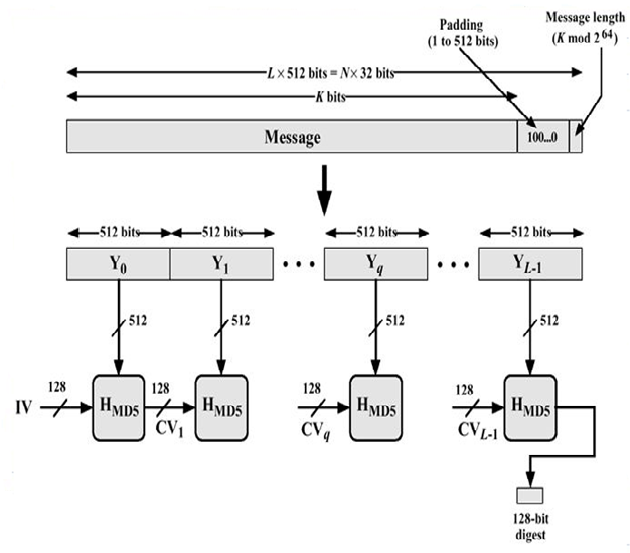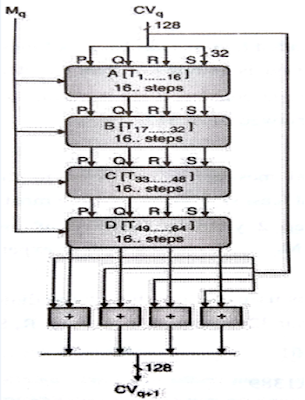## Thursday, October 28, 2021

### Message Digest 5 algorithm (MD 5 algorithm) | Working of MD 5 | How message digest generate using MD5 algorithm

Introduction

128-bit message digest developed by Ron Rivest. This algorithm takes the input length of arbitrary length and 128-bit message digest is produced. The input message is 512-bit blocks. Figure shows processing of message to produce message digest.Figure: Message Digest generation using MD5

MD 5 Algorithm

Step 1: Append Padding Bits – The message is padded to make the length of message is 448 mod 512. 64 bits is padded with 448 bits and convert into multiple of 512 bits. The padding message consists a single 1-bit followed by 0 bits. The length of padding bits is in between 1 to 512.

Step 2: Append Length – 64 bit of original message is appended to the result of above step 1. It is appended such that least significant bytes to most significant byte. The output of step 2 yields a message of integer multiple of 512 bits. As M0, M1,..., Mq,..., ML-1. The total length of expended message is L * 512 bits.Figure: Intialized MD Buffer

Step 3: Initialize MD Buffer – A 128-bit buffer is used to store the intermediate as well as final result. A buffer is represented as four 32-bit registers as four 32-bit registers as P, Q, R, S.

P = 01 23 45 67

Q = 89 AB CD EF

R = FE DC BA 98

S = 76 54 32 10

It is used an initial value (IV).

Step 4: Process Message in 512-bit blocks It consists of four rounds of processing as shown in figure. These four rounds have similar structure as SHA but differ in primitive logical function referred as A, B, C, D. Each round takes input 512-bit block, processed it and produces 128-bit output. The output of fourth round is added to the first round CVq to produce CVq+1.

Step 5: Output – After processing all L 512-bit blocks, the 128-bit message digest is produced as an output.